Get inspired by the success stories of our students in IIT JAM MS, ISI  MStat, CMI MSc DS.  Learn More

# ISI MStat PSB 2013 Problem 4 | Linear RegressionThis is a sample problem from ISI MStat PSB 2013 Problem 4. It is based on the simple linear regression model, finding the estimates, and MSEs. But think over the "Food for Thought" any kind of discussion will be appreciated. Give it a try!

## Problem- ISI MStat PSB 2013 Problem 4

Consider n independent observation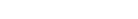from the model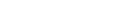,

whereis normal with mean 0 and variance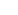. Let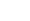andbe the maximum likelihood estimators of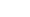and, respectively. Let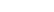and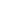be the estimated values of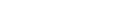and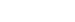, respectively.

(a) What is the estimated mean of Y, when when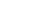? Estimate the mean squared error of this estimator .

(b) What is the predicted value of Y, when when? Estimate the mean squared error of this predictor .

### Prerequisites

Linear Regression

Method of Least Squares

Maximum likelihood Estimators.

Mean Squared Error.

## Solution :

Here for the given model,

we have , the random errors,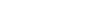, and the maximum likelihood estimators (MLE), of the model parameters are given byand. The interesting thing about this model is, since the random errorsare Gaussian Random variables, the Ordinary Least Square Estimates of the model parametersand, are identical to their Maximum Likelihood Estimators, ( which are already given!). How ?? Verify it yourself and once and remember it henceforth.

So, hereandthere also the OLS estimates of the model parameters respectively.

And By Gauss-Markov Theorem, the OLS estimates of the model parameters are the BLUE (Best Linear Unbiased Estimator), for the model parameters. So, hereandare also the unbiased estimators ofandrespectively.

(a) Now we need to find the estimated mean Y given,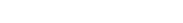is the estimated mean of Y given.

Now since, the given MLEs ( OLSEs) are also unbiased for their respective parameters,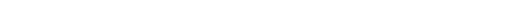=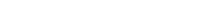=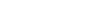=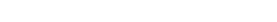So,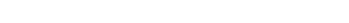.

(b) Similarly, when, the predicted value of Y would be,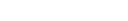is the predicted value of Y whenis given.

Using similar arguments, as in (a) Properties of independence between the model parameters , verify that,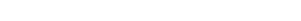. Hence we are done !

## Food For Thought

Now, can you explain Why, the Maximum Likelihood Estimators and Ordinary Least Square Estimates are identical, when the model assumes Gaussian errors ??

Wait!! Not done yet. The main course is served below !!

In a game of dart, a thrower throws a dart randomly and uniformly in a unit circle. Let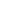be the angle between the line segment joining the dart and the center and the horizontal axis, now consider Z be a random variable. When the thrower is lefty , Z=-1 and when the thrower is right-handed , Z=1 . Assume that getting a Left-handed and Right-handed thrower is equally likely ( is it really equally likely, in real scenario ?? ). Can you construct a regression model, for regressingon Z.

Think over it, if you want to discuss, we can do that too !!

## Subscribe to Cheenta at Youtube

This is a sample problem from ISI MStat PSB 2013 Problem 4. It is based on the simple linear regression model, finding the estimates, and MSEs. But think over the "Food for Thought" any kind of discussion will be appreciated. Give it a try!

## Problem- ISI MStat PSB 2013 Problem 4

Consider n independent observationfrom the model,

whereis normal with mean 0 and variance. Letandbe the maximum likelihood estimators ofand, respectively. Letandbe the estimated values ofand, respectively.

(a) What is the estimated mean of Y, when when? Estimate the mean squared error of this estimator .

(b) What is the predicted value of Y, when when? Estimate the mean squared error of this predictor .

### Prerequisites

Linear Regression

Method of Least Squares

Maximum likelihood Estimators.

Mean Squared Error.

## Solution :

Here for the given model,

we have , the random errors,, and the maximum likelihood estimators (MLE), of the model parameters are given byand. The interesting thing about this model is, since the random errorsare Gaussian Random variables, the Ordinary Least Square Estimates of the model parametersand, are identical to their Maximum Likelihood Estimators, ( which are already given!). How ?? Verify it yourself and once and remember it henceforth.

So, hereandthere also the OLS estimates of the model parameters respectively.

And By Gauss-Markov Theorem, the OLS estimates of the model parameters are the BLUE (Best Linear Unbiased Estimator), for the model parameters. So, hereandare also the unbiased estimators ofandrespectively.

(a) Now we need to find the estimated mean Y given,is the estimated mean of Y given.

Now since, the given MLEs ( OLSEs) are also unbiased for their respective parameters,===So,.

(b) Similarly, when, the predicted value of Y would be,is the predicted value of Y whenis given.

Using similar arguments, as in (a) Properties of independence between the model parameters , verify that,. Hence we are done !

## Food For Thought

Now, can you explain Why, the Maximum Likelihood Estimators and Ordinary Least Square Estimates are identical, when the model assumes Gaussian errors ??

Wait!! Not done yet. The main course is served below !!

In a game of dart, a thrower throws a dart randomly and uniformly in a unit circle. Letbe the angle between the line segment joining the dart and the center and the horizontal axis, now consider Z be a random variable. When the thrower is lefty , Z=-1 and when the thrower is right-handed , Z=1 . Assume that getting a Left-handed and Right-handed thrower is equally likely ( is it really equally likely, in real scenario ?? ). Can you construct a regression model, for regressingon Z.

Think over it, if you want to discuss, we can do that too !!

## Subscribe to Cheenta at Youtube

This site uses Akismet to reduce spam. Learn how your comment data is processed.

### Knowledge Partner